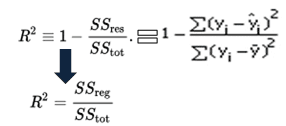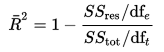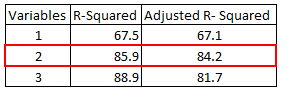# Difference between Adjusted R-squared and R-squared

In this tutorial, we will cover the difference between r-squared and adjusted r-squared. It includes detailed theoretical and practical explanation of these two statistical metrics in R.

R-squared (R²)

It measures the proportion of the variation in your dependent variable explained by all of your independent variables in the model. It assumes that every independent variable in the model helps to explain variation in the dependent variable. In reality, some independent variables (predictors) don't help to explain dependent (target) variable. In other words, some variables do not contribute in predicting target variable.

Mathematically, R-squared is calculated by dividing sum of squares of residuals (SSres) by total sum of squares (SStot) and then subtract it from 1. In this case, SStot measures total variation. SSreg measures explained variation and SSres measures unexplained variation.

As SSres + SSreg = SStot, R² = Explained variation / Total VariationR-squared Equation
R-Squared is also called coefficient of determination. It lies between 0% and 100%. A r-squared value of 100% means the model explains all the variation of the target variable. And a value of 0% measures zero predictive power of the model. Higher R-squared value, better the model.

It measures the proportion of variation explained by only those independent variables that really help in explaining the dependent variable. It penalizes you for adding independent variable that do not help in predicting the dependent variable.

Adjusted R-Squared can be calculated mathematically in terms of sum of squares. The only difference between R-square and Adjusted R-square equation is degree of freedom.Adjusted R-Squared Equation
In the above equation, dft is the degrees of freedom n– 1 of the estimate of the population variance of the dependent variable, and dfe is the degrees of freedom n – p – 1 of the estimate of the underlying population error variance.

Adjusted R-squared value can be calculated based on value of r-squared, number of independent variables (predictors), total sample size.Adjusted R-Squared Equation 2

Difference between R-square and Adjusted R-square
1. Every time you add a independent variable to a model, the R-squared increases, even if the independent variable is insignificant. It never declines. Whereas Adjusted R-squared increases only when independent variable is significant and affects dependent variable.

2. In the table below, adjusted r-squared is maximum when we included two variables. It declines when third variable is added. Whereas r-squared increases when we included third variable. It means third variable is insignificant to the model.R-Squared vs. Adjusted R-Squared
3. Adjusted r-squared can be negative when r-squared is close to zero.
4. Adjusted r-squared value always be less than or equal to r-squared value.
Which is better?
Adjusted R-square should be used to compare models with different numbers of independent variables. Adjusted R-square should be used while selecting important predictors (independent variables) for the regression model.

R : Calculate R-Squared and Adjusted R-Squared

Suppose you have actual and predicted dependent variable values. In the script below, we have created a sample of these values. In this example, y refers to the observed dependent variable and yhat refers to the predicted dependent variable.
y = c(21, 21, 22.8, 21.4, 18.7, 18.1, 14.3, 24.4, 22.8, 19.2)
yhat = c(21.5, 21.14, 26.1, 20.2, 17.5, 19.7, 14.9, 22.5, 25.1, 18)
R.squared = 1 - sum((y-yhat)^2)/sum((y-mean(y))^2)
print(R.squared)
Final Result : R-Squared = 0.6410828

Let's assume you have three independent variables in this case.
n = 10
p = 3
adj.r.squared = 1 - (1 - R.squared) * ((n - 1)/(n-p-1))
In this case, adjusted r-squared value is 0.4616242 assuming we have 3 predictors and 10 observations.

## Python : Calculate Adjusted R-Squared and R-Squared

import numpy as np
y = np.array([21, 21, 22.8, 21.4, 18.7, 18.1, 14.3, 24.4, 22.8, 19.2])
yhat = np.array([21.5, 21.14, 26.1, 20.2, 17.5, 19.7, 14.9, 22.5, 25.1, 18])
R2 = 1 - np.sum((yhat - y)**2) / np.sum((y - np.mean(y))**2)
R2
n=y.shape
p=3
adj_rsquared = 1 - (1 - R2) * ((n - 1)/(n-p-1))

data temp;
input y yhat;
cards;
21 21.5
21 21.14
22.8 26.1
21.4 20.2
18.7 17.5
18.1 19.7
14.3 14.9
24.4 22.5
22.8 25.1
19.2 18
;
run;

data out2;
set temp ;
d=y-yhat;
absd=abs(d);
d2 = d**2;
run;

/* Residual Sum of Square */
proc means data = out2 ;
var d2;
run;

data _null_;
run;

/* Total Sum of Square */
proc means data = temp ;
var y;
output out=avg_y mean=avg_y;
run;

data _null_;
set avg_y;
call symputx ('avgy', avg_y);
run;

%put &avgy.;

data out22;
set temp ;
diff = y - &avgy.;
diff2= diff**2;
run;

proc means data = out22 ;
var diff2;
output out=TSS sum=;
run;

data _null_;
set TSS;
call symputx ('TSS', diff2);
run;

/* Calculate the R2 */
%put &RSQ;

/* Calculate the Adj R2 */
%LET N = 10;
%LET P = 3;Share
Related PostsDeepanshu founded ListenData with a simple objective - Make analytics easy to understand and follow. He has over 10 years of experience in data science. During his tenure, he has worked with global clients in various domains like Banking, Insurance, Private Equity, Telecom and Human Resource.

9 Responses to "Difference between Adjusted R-squared and R-squared"
1.Could you please explain RMSE, AIC and BIC as well.
We use RMSE to compare our model. Lesser the value is good for our model, but I m not sure about the rest of the statistics AIC and BIC respectively..

2.Nicely Explained

3.nice explaination so it means always adjusted r square will <= Rsquare

4.Could you give please the data set in order to understand the difference better.

5.R squared depends on the sum of squared errors (SSE), il SSE decreases (new predictor has improved the fit) then R squared increases. In this case R squared is a good measure. Please give us a complete example to understand. Thank you

6.its really good effort to explain it.. Also explain BIC and AIC

7.nicely explained

8.Good explanation

9.Next → ← Prev